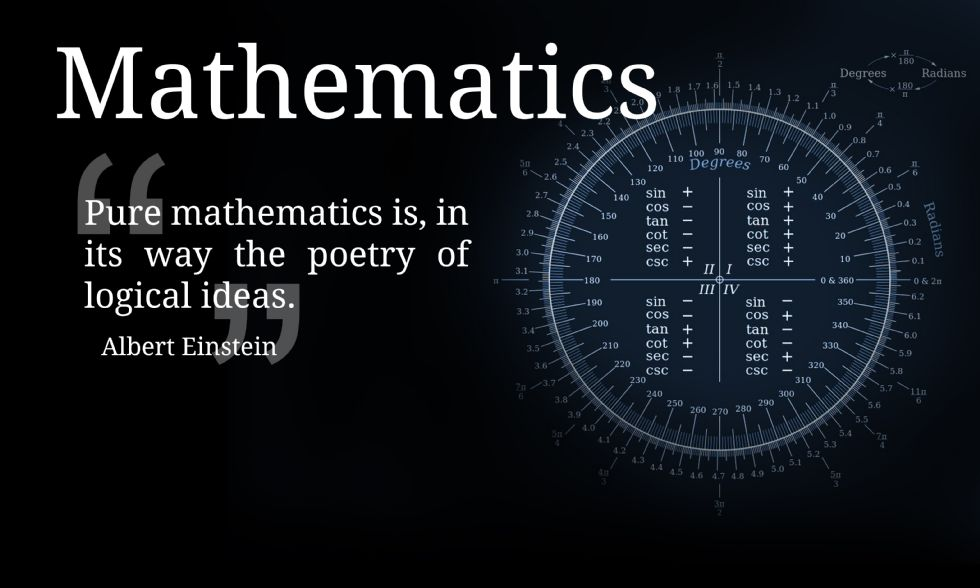Skip to content ↓

Water Orton Road, Castle Bromwich, Birmingham. B36 9HF

Telephone:0121 748 0400

Fax:0121 748 0414

Contact:Mr Cox

# Year 9### Year 9 Foundation

 Assessment Cycle Topics covered 1 Place value Four operations BIDMAS Rounding and estimation Squares, cubes, roots and other powers Index notation and laws Factors, multiples and primes Lowest Common Multiples and Highest Common Factor Writing algebraic expressions Simplifying algebra Expanding brackets Factorising expressions Substitution 2 Data collection sheets Two way tables Time series Time Coordinates Types of graph Stem and leaf diagrams Pie Charts Scatter graphs Fractions, Decimals and Percentages Four operations with fractions 3 Percentages of amounts Form and solve equations Solve equations graphically Inequalities Solve inequalities Represent inequalities pictorially Function machines Sequences Nth term Continue quadratic sequences Angle rules Angles in parallel lines Summer Angles in polygons Types of data Sampling Averages Averages from a table

### Year 9 Higher

 Assessment Cycle Topics covered 1 Four operations Rounding and estimation Index laws, including fractional and negative Factors, multiples and primes HCF and LCM Standard form Simplifying surds Substitution Expanding and factorising single brackets Expanding and factorising quadratics 2 Form and solve equations Change the subject of a formula Iteration Proof Linear sequences Nth term Quadratic sequences Nth term of a quadratic sequence Arithmetic and geometric sequences Two way tables Averages Averages from a frequency table, both grouped and discrete Draw and interpret pie charts Time-series graphs Frequency polygons Scatter graphs 3 Fractions, decimals and percentages Add,  subtract, multiply and divide fractions Recurring decimals Percentage of an amount Percentage increase and decrease Compound interest Reverse percentages Sharing in a given ratio Representing ratio as a fraction Recipes Conversion graphs Angle rules Angles in parallel lines Angles ion polygons Pythagoras’ Theorem Trigonometry Summer Coordinates Distance-time/Velocity-time graphs Midpoints of line segments Length of line segments Equation of a line segment